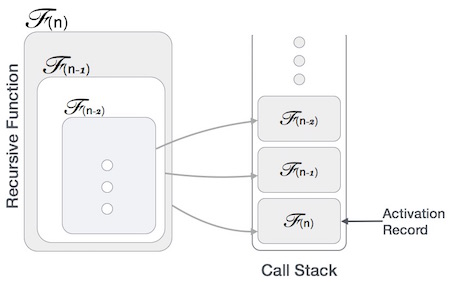# Recursion Algorithms

## Recursion

Some computer programming languages allow a module or function to call itself. This technique is known as recursion. In recursion, a function α either calls itself directly or calls a function β that in turn calls the original function α. The function α is called recursive function.

Example − a function calling itself.

int function(int value) {
if(value < 1)
return;
function(value - 1);

printf("%d ",value);
}


Example − a function that calls another function which in turn calls it again.

int function1(int value1) {
if(value1 < 1)
return;
function2(value1 - 1);
printf("%d ",value1);
}
int function2(int value2) {
function1(value2);
}


## Properties

A recursive function can go infinite like a loop. To avoid infinite running of recursive function, there are two properties that a recursive function must have −

• Base criteria − There must be at least one base criteria or condition, such that, when this condition is met the function stops calling itself recursively.

• Progressive approach − The recursive calls should progress in such a way that each time a recursive call is made it comes closer to the base criteria.

## Implementation

Many programming languages implement recursion by means of stacks. Generally, whenever a function (caller) calls another function (callee) or itself as callee, the caller function transfers execution control to the callee. This transfer process may also involve some data to be passed from the caller to the callee.

This implies, the caller function has to suspend its execution temporarily and resume later when the execution control returns from the callee function. Here, the caller function needs to start exactly from the point of execution where it puts itself on hold. It also needs the exact same data values it was working on. For this purpose, an activation record (or stack frame) is created for the caller function.This activation record keeps the information about local variables, formal parameters, return address and all information passed to the caller function.

## Analysis of Recursion

One may argue why to use recursion, as the same task can be done with iteration. The first reason is, recursion makes a program more readable and because of latest enhanced CPU systems, recursion is more efficient than iterations.

## Time Complexity

In case of iterations, we take number of iterations to count the time complexity. Likewise, in case of recursion, assuming everything is constant, we try to figure out the number of times a recursive call is being made. A call made to a function is Ο(1), hence the (n) number of times a recursive call is made makes the recursive function Ο(n).

## Space Complexity

Space complexity is counted as what amount of extra space is required for a module to execute. In case of iterations, the compiler hardly requires any extra space. The compiler keeps updating the values of variables used in the iterations. But in case of recursion, the system needs to store activation record each time a recursive call is made. Hence, it is considered that space complexity of recursive function may go higher than that of a function with iteration.

## Example

Following are the implementations of the recursion in various programming languages −

// C program for Recursion Data Structure
#include <stdio.h>
int factorial(int n) {
// Base case: factorial of 0 is 1
if (n == 0)
return 1;
// Recursive case: multiply n with factorial of (n-1)
return n * factorial(n - 1);
}
int main() {
// case 1
int number = 6;
printf("Number is: %d\n" , 6);
//case 2
if (number < 0) {
printf("Error: Factorial is undefined for negative numbers.\n");
return 1;
}
int result = factorial(number);
//print the output
printf("Factorial of %d is: %d\n", number, result);
return 0;
}


### Output

Number is: 6
Factorial of 6 is: 720

// CPP program for Recursion Data Structure
#include <iostream>
int factorial(int n) {
// Base case: factorial of 0 is 1
if (n == 0)
return 1;
// Recursive case: multiply n with factorial of (n-1)
return n * factorial(n - 1);
}
int main() {
// case 1
int number = 6;
std::cout<<"Number is: "<<number<<"\n";
//case 2
if (number < 0) {
std::cout << "Error: Factorial is undefined for negative numbers.\n";
return 1;
}
int result = factorial(number);
//print the output
std::cout << "Factorial of " << number << " is: " << result << std::endl;
return 0;
}


### Output

Number is:  6
Factorial of 6 is: 720

// Java program for Recursion Data Structure
import java.util.Scanner;
public class Main {
public static int factorial(int n) {
// Base case: factorial of 0 is 1
if (n == 0)
return 1;
// Recursive case: multiply n with factorial of (n-1)
return n * factorial(n - 1);
}
public static void main(String[] args) {
//Case 1
int number = 6;
System.out.println("Number is: " + number);
//Case 2
if (number < 0) {
System.out.println("Error: Factorial is undefined for negative numbers.");
System.exit(1);
}
int result = factorial(number);
//print the output
System.out.println("Factorial of " + number + " is: " + result);
}
}


### Output

Number is: 6
Factorial of 6 is: 720

# Python program for Recursion Data Structure
def factorial(n):
#Base Case: factorial of 0 is 1
if n == 0:
return 1
# Recursive case: multiply n with factorial of (n-1)
return n * factorial(n - 1)
#Case 1:
number = 6;
print("Number is: ", number);
#Case 2:
if number < 0:
print("Error: Factorial is undefined for negative numbers.")
else:
result = factorial(number)
# print the output
print("Factorial of", number, "is: ", result)


### Output

Number is:  6
Factorial of 6 is: 720